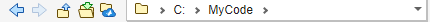# pwd

## 语法

``pwd``
``currentFolder = pwd``

## 说明

``pwd` 返回 MATLAB® 当前文件夹。`

``currentFolder = pwd` 返回当前文件夹的路径。`

## 示例

```cd c:\myMATLABFiles currentFolder = pwd ```
```currentFolder = 'c:\myMATLABFiles' ```
• 在“当前文件夹”工具栏中查看当前文件夹。## 输出参数

MATLAB 当前文件夹路径，以字符向量形式返回。